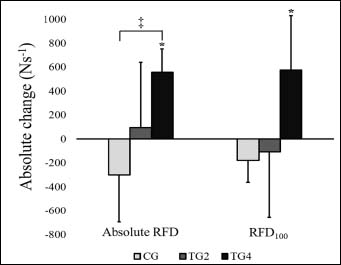Figure 4. Absolute change in rate of force development (RFD (Ns-1)). Absolute RFD refers to the RFD calculated from the onset of force to the maximal force, while RFD100 refers to the RFD calculated as the rise in force in the first 100 milliseconds of contraction. * = significant change from pre-test (p < 0.05). ‡ = significant difference in change between groups (p < 0.05)